# Sum Values in a Column based on Criteria in Another Column

This post will guide you how to sum values in a column based on criteria in another column in Excel. How do I sum values based on criteria in another column with Pivot table in Excel. How to sum all values in one column based on the text value in another column with formula in Excel.

Assuming that you have a list of data set in range A1:B6 and you want to sum the values in one column (Column B) based on the text value in another column (Column A), how to achieve it. You need to remove duplicates values in Column a, then sum values in column B based on the unique text values in column A. You can use the below two methods to achieve the result.

## Sum Values based on Another Column with Formula

To use a formula to sum values in Column B based on Column A, you can create a formula based on the SUMIF function. Just do the following steps:

#1 select the text values in Column A (A1:A6), press Ctrl +C to copy these values, and paste into another blank column (Column D).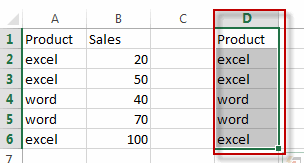#2 keep the pasted values in Column D selected, go to DATA tab, click Remove Duplicates command under Data Tools group.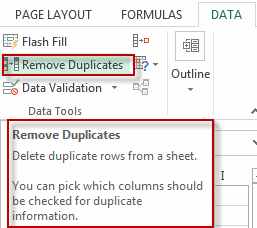#3 select only pasted column in columns list under Remove Duplicates dialog box. And click Ok button.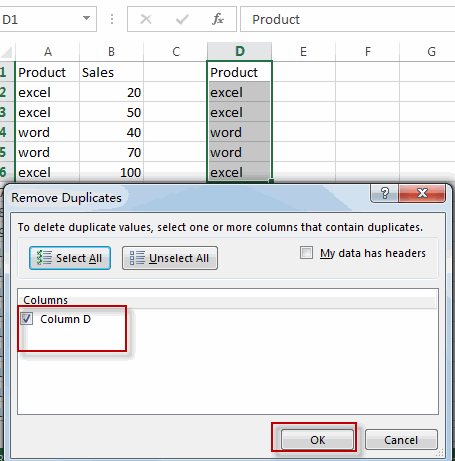Note: the duplicated values in selected Column D have been removed and only keep unique values.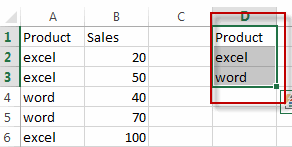#4 select one blank cell (E2) beside the pasted column and then type the following formula into the formula box and press Enter key in your keyboard, and drag the AutoFill Handle over other cells.

`=SUMIF(\$A\$2:\$A\$6, D2, \$B\$2:\$B\$6)`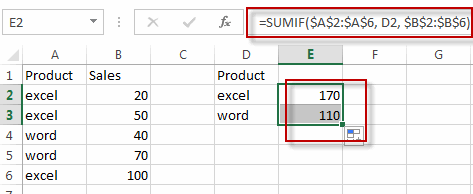You will see that the summed values have been calculated in column E based on the text values in Column A.

## Sum Values based on Another Column with Pivot Table

You can also create an Excel Pivot Table to sum values based on another column. Just do the following steps:

#1 select the data source that to be used for creating PivotTable.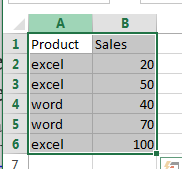#2 go to INSERT tab, click PivotTable command under Tables group. The Create PivotTable dialog box will open.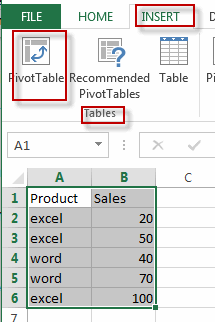#3 select the Existing Worksheet radio button, and select one range of cells that used to place the pivot table.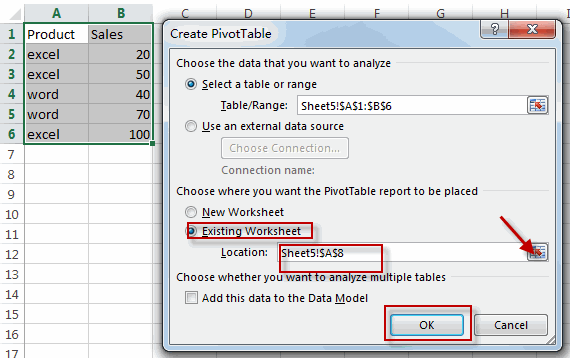#4 Choose fields to add to report in the PivotTable Fields pane. Drag the column contain text values into the ROWS section, and drag the column contain sale values into the VALUES section.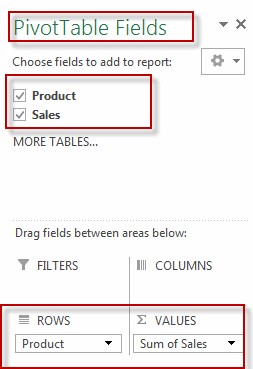#5 the values in column B based on another column A have been summed.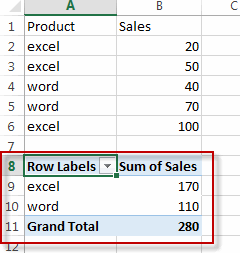### Related Functions

• Excel SUMIF Function
The Excel SUMIF function sum the numbers in the range of cells that meet a single criteria that you specify. The syntax of the SUMIF function is as below:=SUMIF (range, criteria, [sum_range])…